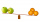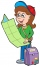# Fraction calculator

The calculator performs basic and advanced operations with fractions, expressions with fractions combined with integers, decimals, and mixed numbers. It also shows detailed step-by-step information about the fraction calculation procedure. Solve problems with two, three, or more fractions and numbers in one expression.

## Result:

### 55/7 * 31/8 = 125/7 = 17 6/7 ≅ 17.8571429

Spelled result in words is one hundred twenty-five sevenths (or seventeen and six sevenths).

### How do you solve fractions step by step?

1. Conversion a mixed number 5 5/7 to a improper fraction: 5 5/7 = 5 5/7 = 5 · 7 + 5/7 = 35 + 5/7 = 40/7

To find a new numerator:
a) Multiply the whole number 5 by the denominator 7. Whole number 5 equally 5 * 7/7 = 35/7
b) Add the answer from previous step 35 to the numerator 5. New numerator is 35 + 5 = 40
c) Write a previous answer (new numerator 40) over the denominator 7.

Five and five sevenths is forty sevenths
2. Conversion a mixed number 3 1/8 to a improper fraction: 3 1/8 = 3 1/8 = 3 · 8 + 1/8 = 24 + 1/8 = 25/8

To find a new numerator:
a) Multiply the whole number 3 by the denominator 8. Whole number 3 equally 3 * 8/8 = 24/8
b) Add the answer from previous step 24 to the numerator 1. New numerator is 24 + 1 = 25
c) Write a previous answer (new numerator 25) over the denominator 8.

Three and one eighth is twenty-five eighths
3. Multiple: 40/7 * 25/8 = 40 · 25/7 · 8 = 1000/56 = 125 · 8/7 · 8 = 125/7
Multiply both numerators and denominators. Result fraction keep to lowest possible denominator GCD(1000, 56) = 8. In the following intermediate step, cancel by a common factor of 8 gives 125/7.
In other words - forty sevenths multiplied by twenty-five eighths = one hundred twenty-five sevenths.

#### Rules for expressions with fractions:

Fractions - simply use a forward slash between the numerator and denominator, i.e., for five-hundredths, enter 5/100. If you are using mixed numbers, be sure to leave a single space between the whole and fraction part.
The slash separates the numerator (number above a fraction line) and denominator (number below).

Mixed numerals (mixed fractions or mixed numbers) write as integer separated by one space and fraction i.e., 1 2/3 (having the same sign). An example of a negative mixed fraction: -5 1/2.
Because slash is both signs for fraction line and division, we recommended use colon (:) as the operator of division fractions i.e., 1/2 : 3.

Decimals (decimal numbers) enter with a decimal point . and they are automatically converted to fractions - i.e. 1.45.

The colon : and slash / is the symbol of division. Can be used to divide mixed numbers 1 2/3 : 4 3/8 or can be used for write complex fractions i.e. 1/2 : 1/3.
An asterisk * or × is the symbol for multiplication.
Plus + is addition, minus sign - is subtraction and ()[] is mathematical parentheses.
The exponentiation/power symbol is ^ - for example: (7/8-4/5)^2 = (7/8-4/5)2

#### Examples:

subtracting fractions: 2/3 - 1/2
multiplying fractions: 7/8 * 3/9
dividing Fractions: 1/2 : 3/4
exponentiation of fraction: 3/5^3
fractional exponents: 16 ^ 1/2
adding fractions and mixed numbers: 8/5 + 6 2/7
dividing integer and fraction: 5 ÷ 1/2
complex fractions: 5/8 : 2 2/3
decimal to fraction: 0.625
Fraction to Decimal: 1/4
Fraction to Percent: 1/8 %
comparing fractions: 1/4 2/3
multiplying a fraction by a whole number: 6 * 3/4
square root of a fraction: sqrt(1/16)
reducing or simplifying the fraction (simplification) - dividing the numerator and denominator of a fraction by the same non-zero number - equivalent fraction: 4/22
expression with brackets: 1/3 * (1/2 - 3 3/8)
compound fraction: 3/4 of 5/7
fractions multiple: 2/3 of 3/5
divide to find the quotient: 3/5 ÷ 2/3

The calculator follows well-known rules for order of operations. The most common mnemonics for remembering this order of operations are:
PEMDAS - Parentheses, Exponents, Multiplication, Division, Addition, Subtraction.
BEDMAS - Brackets, Exponents, Division, Multiplication, Addition, Subtraction
BODMAS - Brackets, Of or Order, Division, Multiplication, Addition, Subtraction.
GEMDAS - Grouping Symbols - brackets (){}, Exponents, Multiplication, Division, Addition, Subtraction.
Be careful, always do multiplication and division before addition and subtraction. Some operators (+ and -) and (* and /) has the same priority and then must evaluate from left to right.

## Fractions in word problems:

• DividendsThe three friends divided the win by the invested money. Karlos got three-eighths, John 320 permille, and the rest got Martin. Who got the most and which the least?
• Equivalent expressionsA coach took his team out for pizza after their last game. There were 14 players, so they had to sit in smaller groups at different tables. Six players sat at one table and got 4 small pizzas to share equally. The other players sat at the different table
• Sort fractionsWhich of the following fractions is the largest? 29/36 5/6 7/9 3/4
• Leo hikedLeo hiked 6/7 of a kilometer. Jericho hiked 2/3 kilometer. Who covered a longer distance? How much longer?
• Math testBrayden was solving some math problems for the math team. He answered 2 math problems. Matthew answered 3, John answered 1 reasoning. Matthew 1/2 times as many. Brayden said that 2/6. Is he correct? Why or why not? Be sure to explain your answer.
• 5 books5 books cost \$28.75. Justin wants to buy 8 similar books. He has \$50. Does he have enough?
• Engineer KažimírThe difference between politicians-demagogues and reasonable person with at least primary education beautifully illustrated by the TV show example. "Engineer" Kažimír says that during their tenure there was a large decline in the price of natural gas, pri
• Ten fractionsWrite ten fractions between 1/3 and 2/3
• Comparing and sortingArrange in descending order this fractions: 2/7, 7/10 & 1/2
• Turtles 2A box turtle hibernates in the sand at 11 5/8. A spotted turtle hibernates at 11 16/25 feet. Which turtle is deeper? Write answer as number 1 or 2.
• ArrangeArrange the following in descending order: 0.32, 2on5, 27%, 1 on 3
• CompareCompare fractions (34)/(3) and (12)/(4). Which fraction of the lower?
• Stones in aquariumIn an aquarium with a length 2 m; width 1.5 m and a depth of 2.5 m is a water level up to three-quarters of the depth. Can we place stones with a volume of 2 m3 into the aquarium without water being poured out?